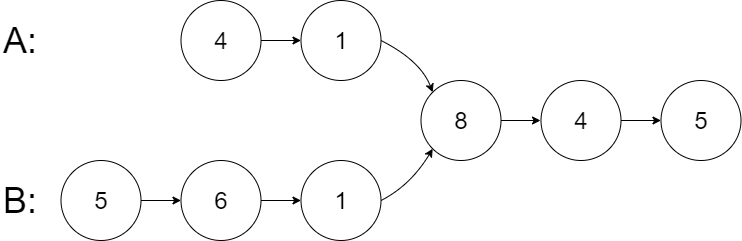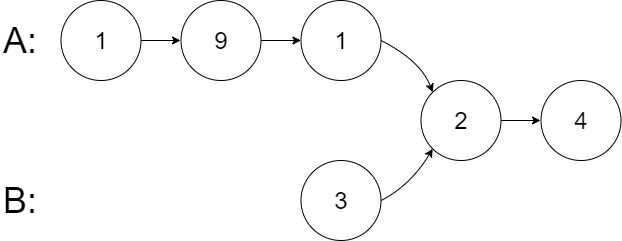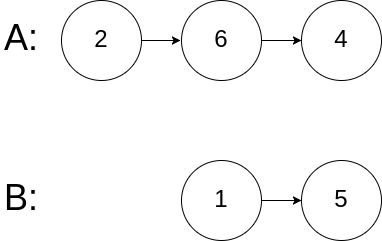# Intersection of Two Linked Lists LeetCode Solution

Difficulty Level Easy
Frequently asked in Adobe Airbnb Amazon Apple Bloomberg ByteDance eBay Facebook Goldman Sachs Google Intuit LinkedIn Microsoft Morgan Stanley Nutanix Nvidia Oracle PayPal Qualcomm Samsung Spotify Uber Yahoo
Redfin tiktokViews 22

## Problem Statement

Intersection of Two Linked Lists LeetCode Solution – We are given the heads of two strongly linked-lists headA and headB. It is also given that the two linked lists may intersect at some point. We are asked to return the node at which they intersect or null if they have no intersection.

## Examples & Explanation

Example 1:```Input: intersectVal = 8, listA = [4,1,8,4,5], listB = [5,6,1,8,4,5], skipA = 2, skipB = 3
Output: Intersected at '8'
Explanation: The intersected node's value is 8 (note that this must not be 0 if the two lists intersect).
From the head of A, it reads as [4,1,8,4,5]. From the head of B, it reads as [5,6,1,8,4,5]. There are 2 nodes before the intersected node in A; There are 3 nodes before the intersected node in B.```

Example 2:```Input: intersectVal = 2, listA = [1,9,1,2,4], listB = [3,2,4], skipA = 3, skipB = 1
Output: Intersected at '2'
Explanation: The intersected node's value is 2 (note that this must not be 0 if the two lists intersect).
From the head of A, it reads as [1,9,1,2,4]. From the head of B, it reads as [3,2,4]. There are 3 nodes before the intersected node in A; There are 1 node before the intersected node in B.```

Example 3:```Input: intersectVal = 0, listA = [2,6,4], listB = [1,5], skipA = 3, skipB = 2
Output: No intersection
Explanation: From the head of A, it reads as [2,6,4]. From the head of B, it reads as [1,5]. Since the two lists do not intersect, intersectVal must be 0, while skipA and skipB can be arbitrary values.
Explanation: The two lists do not intersect, so return null.```

## Approach

The idea is very intuitive, we can simply find the difference between the lengths of given linked lists and change the root or the head node of the longer list to this difference. Keep iterating the nodes till an intersection is encountered. If one of the nodes becomes NULL or nullptr, then there exists no intersection, return NULL, otherwise return node.

## Code

### C++ code for Intersection of Two Linked Lists

```/**
* struct ListNode {
*     int val;
*     ListNode *next;
*     ListNode(int x) : val(x), next(NULL) {}
* };
*/
class Solution {
public:
// declaring integers to store the size of the linked lists
int a=0, b=0;
// to count the size of the linked list iterate through each node & increment size
while(currA != NULL){
currA = currA -> next;
a++;
}
while(currB != NULL) {
currB = currB->next;
b++;
}

int k = abs(b-a);
if ( b > a ) {
//if the size of B > A, then b becomes b->next till the difference a-b becomes zero
while(k--) {
}
}
else {
//if the size of B < A, then a becomes a->next till the difference a-b becomes zero
while(k--) {
}
}

}

// if one of the list becomes NULL and still no node is found common then return NULL

// if above statement is not true, then return the common node either headA or headB
}
};```

### Java code for Intersection of Two Linked Lists

```/**
* public class ListNode {
*     int val;
*     ListNode next;
*     ListNode(int x) {
*         val = x;
*         next = null;
*     }
* }
*/
public class Solution {
int a=0, b=0;
while(currA != null){
currA = currA.next;
a++;
}
while(currB != null) {
currB = currB.next;
b++;
}
int k = Math.abs(b-a);
if ( b > a ) {
while(k>0) {
k--;
}
}
else {
while(k>0) {
k--;
}
}

}

// if above statement is not true, then return the common node either headA or headB